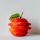Home
IT Knowledge
Inspiration
Languages
EN

# Python - format strings

0 points
Created by:Khloe-Kaiser
398

In this article, we would like to show you how to format strings in Python.

Quick solution:

``````color = "red"
text = "The apple is {}."

print(text.format(color))  # The apple is red.``````

## Practical examples

### Example 1

In this example, to format the string we use `format()` method that takes the passed arguments and puts them in the place of the placeholders `{}`.

``````name = "Tom"
age = 25

text = "This is my friend {}, he is {}."

print(text.format(name, age))``````

Output:

``This is my friend Tom, he is 25.``

### Example 2

You can also specify the placeholders order by using numbered indexes (`{0}`, `{1}`) or named indexes (`{name}`, `{age}`).

``````name = "Tom"
age = 25

text = "This is my friend {1}, he is {0}."

print(text.format(age, name))``````

or

``````text = "This is my friend {name}, he is {age}."

print(text.format(name="Tom", age=25))``````

Output:

``This is my friend Tom, he is 25.``